Translation math

Translation math

In the 19th century, Felix Klein proposed a new perspective on geometry known as transformational geometry. Most of the proofs in geometry are based on the transformations of objects.

There are four types of transformations possible for a graph of a function. They are:

• Rotations
• Translations
• Reflections
• Dilation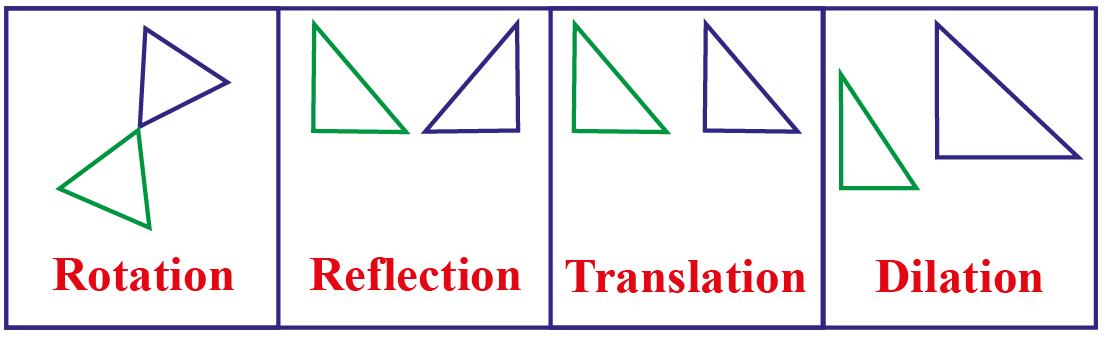In this chapter, we will learn about translation in mathematics using translation math definitions and translation math examples.

Do try your hand at a few translation math problems on translation geometry at the end of the page.

Lesson Plan

 1 What Is Translation? 2 Important Notes on Translation 3 Challenging Questions on Translation 4 Solved Examples on Translation 5 Interactive Questions on Translation

What Is Translation?

Translation Math: Definition

The displacement of a figure or a shape from one place to another is called translation, which means a figure can be moved upward, downward, left, right, or anywhere in the coordinate system using translation.

Translation only involves alteration in the position of the object and hence, there are no changes to the size of the object.

The direction or the path of this change in position of the object can vary i.e., initially the object can move left, then turn right, and so on.

While translating, all the points will shift by the same number of units.

If one point shifts 2 units to the left, then all the points will move 2 units to the left.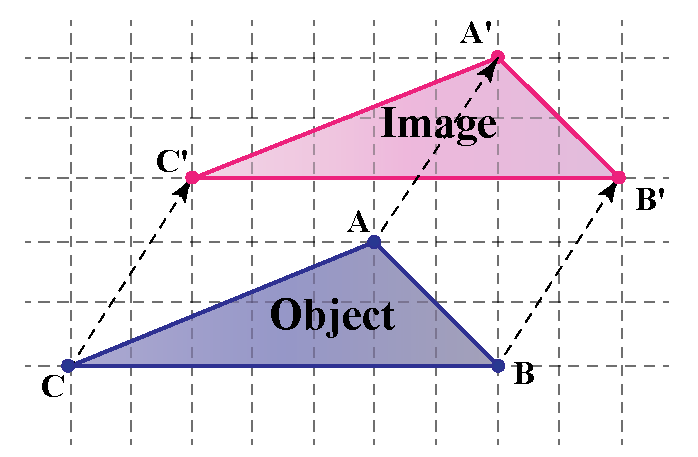Let us look at the following example: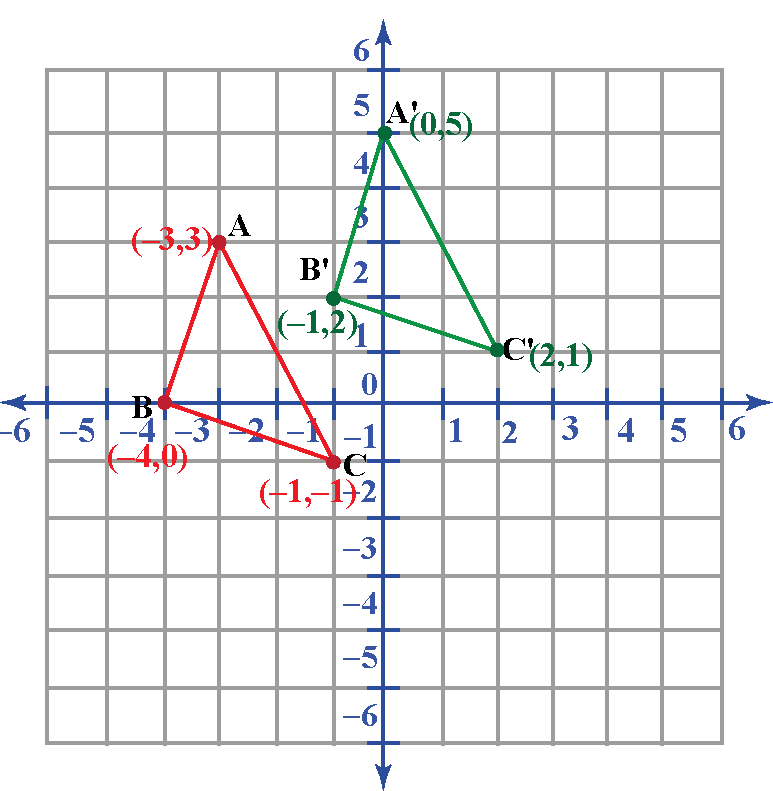In the above example, we see the translation of the given object to the right.

While translating the triangle to the right, we move all the points of the triangle by an equal number of units in the same direction.

What Is the Formula for Translation?

Translation Math: Formula

The translation math formula for any function, $$f(x)$$ is given by:

$$f(x) = f(x + k) + C$$

where,

k = Number of units for translation in x-axis

C = Number of units for translation in y-axis

or,

Any function f(x,y), after translation can be represented as: $$f(x \pm k, y \pm l)$$

 $$f(x) = f(x \pm k) \pm C$$

Translations on the Coordinate Plane

Any object represented in the coordinate plane can be translated horizontally or vertically.

Let us look at the following example to understand translations on the coordinate plane.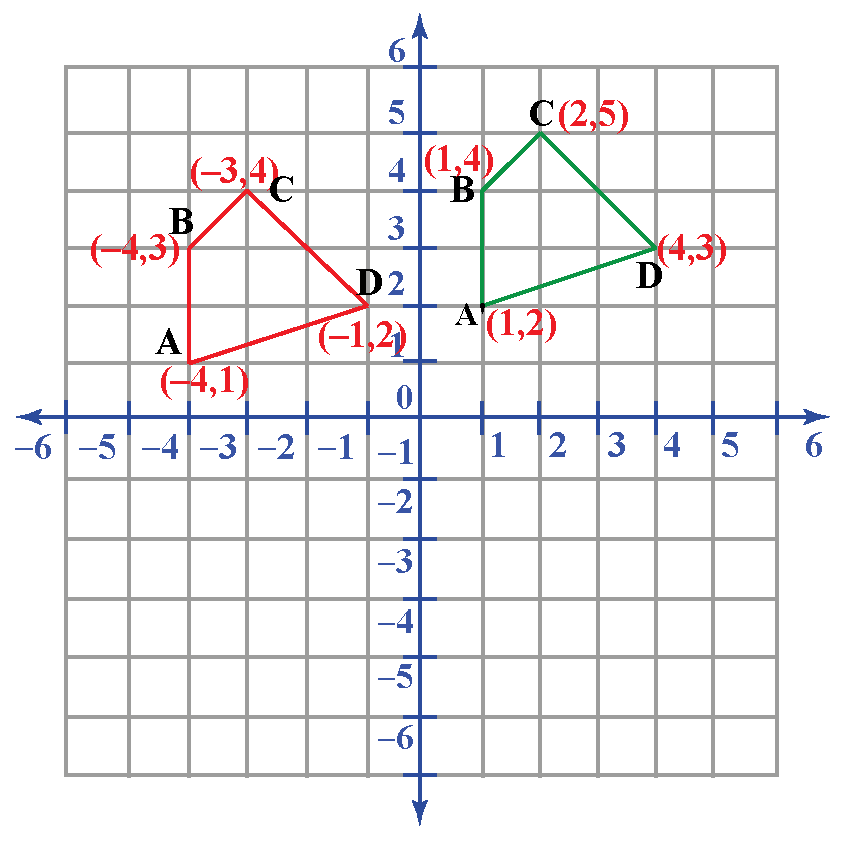A graph is represented in the coordinate plane as shown in the figure.

While translating, the quadrilateral is shifted 5 units horizontally to the right and 1 unit vertically upward, which means the new translated function for the given figure would be

f'(x,y) = f(x - 5, y + 1)

Pre Images and Images

Pre-image is defined as the image of the object on the coordinate plane before translation.

The object after translation is referred to as an image.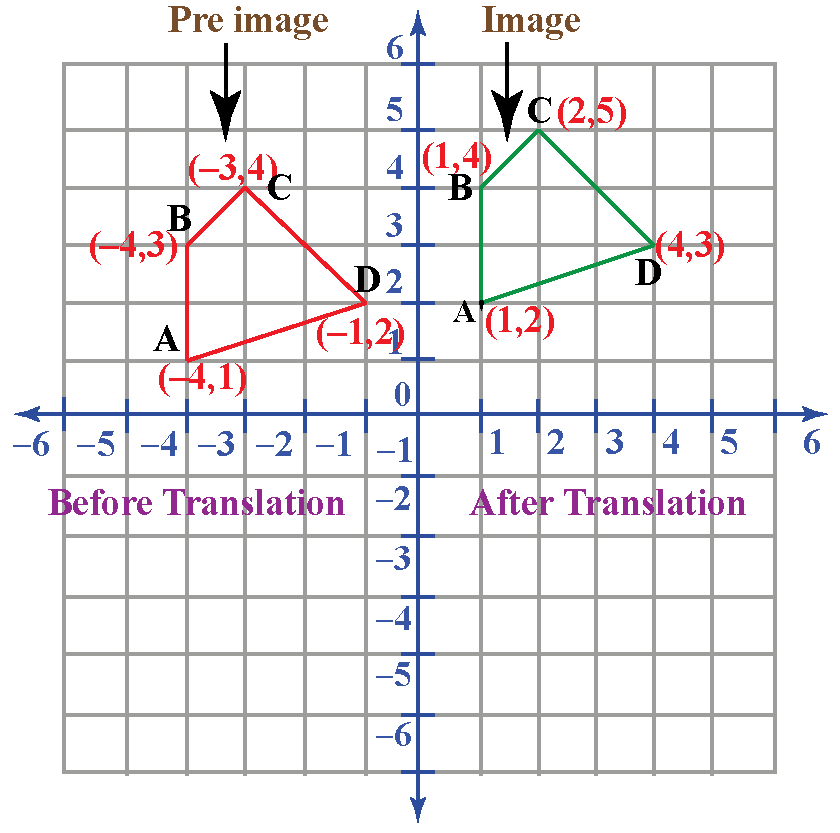Translation Represented by Column Vector or Matrix

Translation Maths: Vector

The translation of any object can be represented by a column vector or column matrix as:

$$\begin{pmatrix} a \\ \end{pmatrix}$$

where, a is the number of units to move right or left along the x-axis and b is the number of units to move up or down along the y-axis.

• The number at the top represents the right or left movement. A positive number means movement to the right and a negative number means movement to the left.
• The number at the bottom represents up and down movement. A positive number means moving up and a negative number means moving down.

In the following figure, triangle ABC is being translated to triangle A’B’C':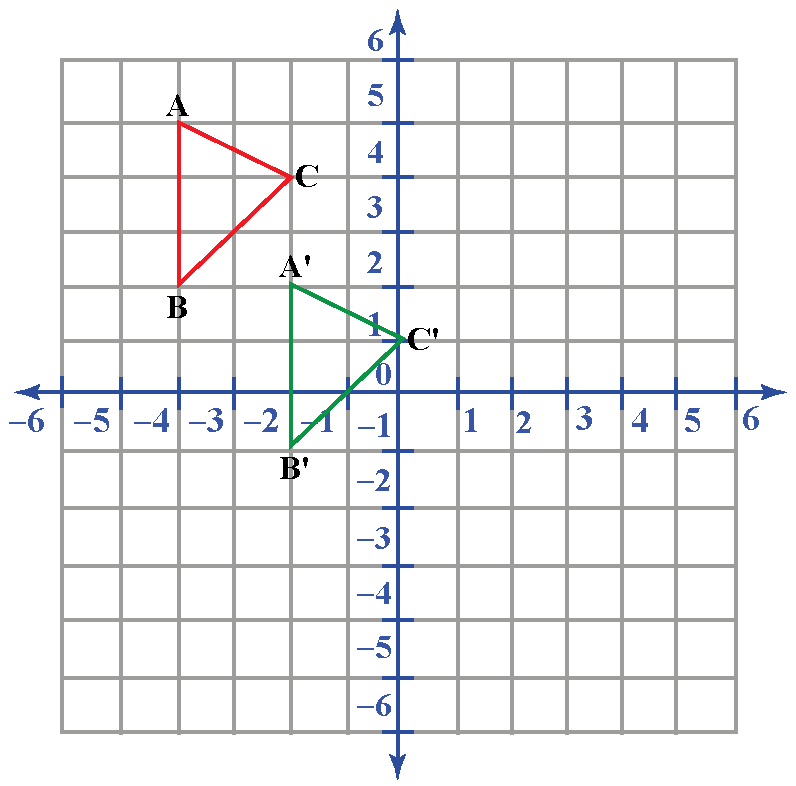The translation math vector for the above example is represented by the column vector:

$$\begin{pmatrix} -2 \\ \end{pmatrix}$$

The basic rules to be followed while translating an object are as follows:

• Positive translation in the x-axis or horizontally would mean shifting to the left, while negative horizontal translation means shifting to the right.
• Positive translation in the y-axis or vertically would mean shifting the object upward while negative vertical translation means shifting downward.
• The shape or size of the object remains unaffected after translation.
• All the points in the object will shift by the same number of units and direction.

How Do You Solve Translations?

Translation for any object in translation geometry can be solved in two sections, depending on whether the object is translated horizontally, vertically, or both.

A function, f(x), after translation can be represented in the general form as:

$$f(x) = f(x \pm k) \pm C$$

where,

• k is the number of units translated horizontally
• C is the number of units translated vertically

Horizontal and vertical translation of an object can be studied in detail in the following section.

Horizontal Translation

While translating horizontally:

• The positive value of k means the object/graph will shift to the left by k units.
• The negative value of k means the object/graph will shift to the right by k units.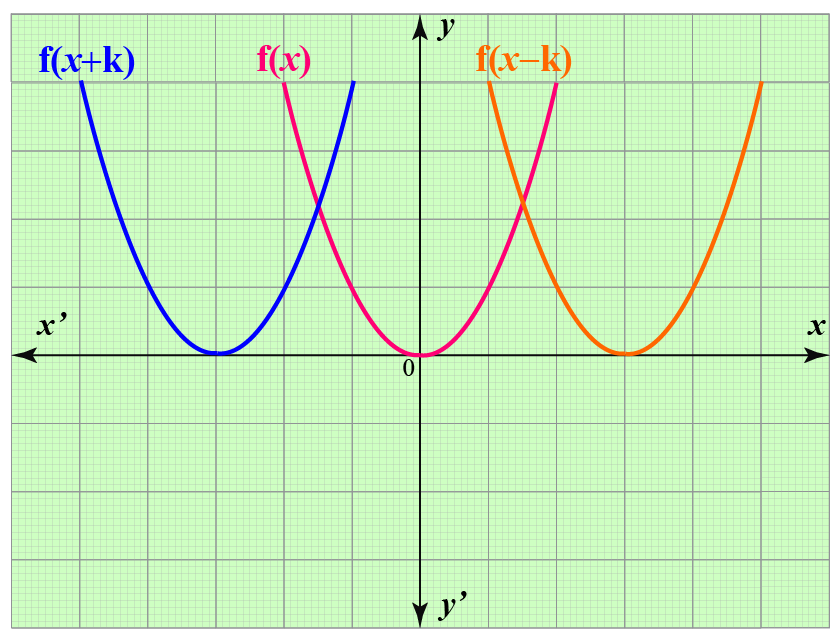Vertical Translation

While translating vertically:

• The positive value of C means the object/graph will shift upward by k units.
• The negative value of C means the object/graph will shift downward by k units.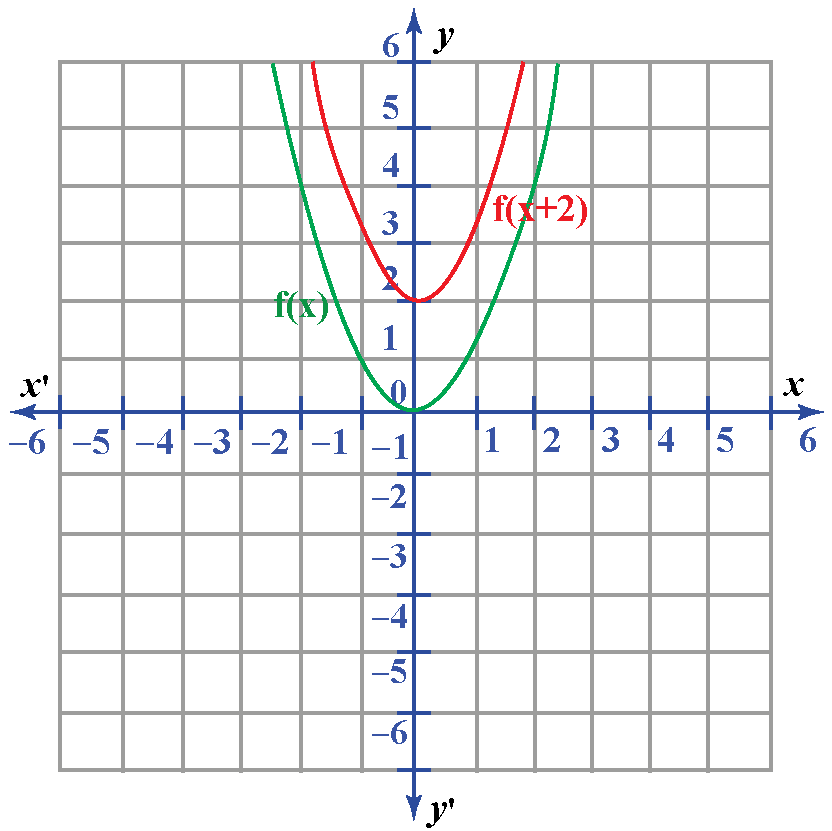The domain of the function remains the same after translation.

How Do You Translate a Shape?

The translation of any shape is similar to the procedure for that of a general object or graph in the coordinate plane.

Translation involves alteration in the position of the shape by a given number of units in the given direction. The size and shape remain unaffected after the translation.

While translating, all the points will shift by the same number of units.

If one point on the shapeshifts by 4 units to the left, then all the points will move 4 units to the left.Challenging Questions
• If the function f(x) has coordinates as (x,y), can you tell what will be coordinates of the point on the curve after C units of vertical translation?
• What are the properties of translation?

Solved Examples

 Example 1

The following graph shows the position of an object before and after translation: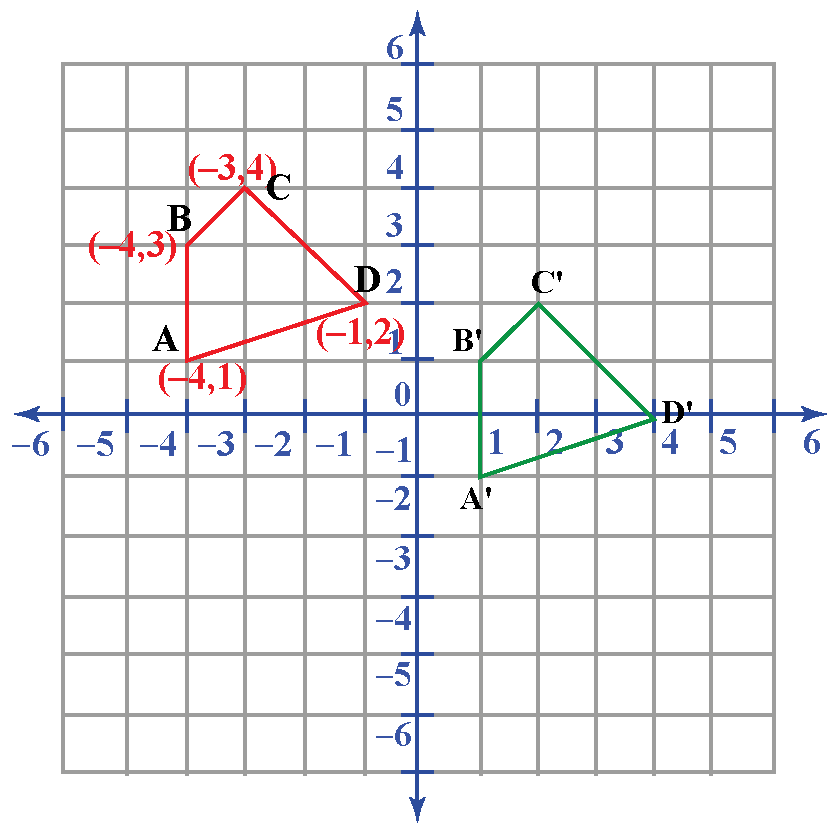Represent the translation of the object in general form from your observations of the graph.

Solution

Coordinates of vertices of the figure are given as:

A = (-4, 1)

B = (-4, 3)

C = (-3, 4)

D = (-1, 2)

Coordinates of the translated figure:

A' =(1, -1)

B' =(1, 1)

C' =(2, 2)

D' =(4, 0)

Thus, comparing the positions of the points of the pre-image and image, we can observe that the object had negative horizontal translation by 5 units and negative vertical translation by 2 units.

For any function f(x, y), the above translation can be represented as f(x-5, y-2).

 Example 2

A triangle with vertices at points (-3, 3), (-4, 0), and (-1, -1) is translated 2 units upward and 3 units toward the right.

Represent this translation on the coordinate plane with pre-image and image.

Solution

The pre-image of the given triangle in the coordinate plane can be drawn as: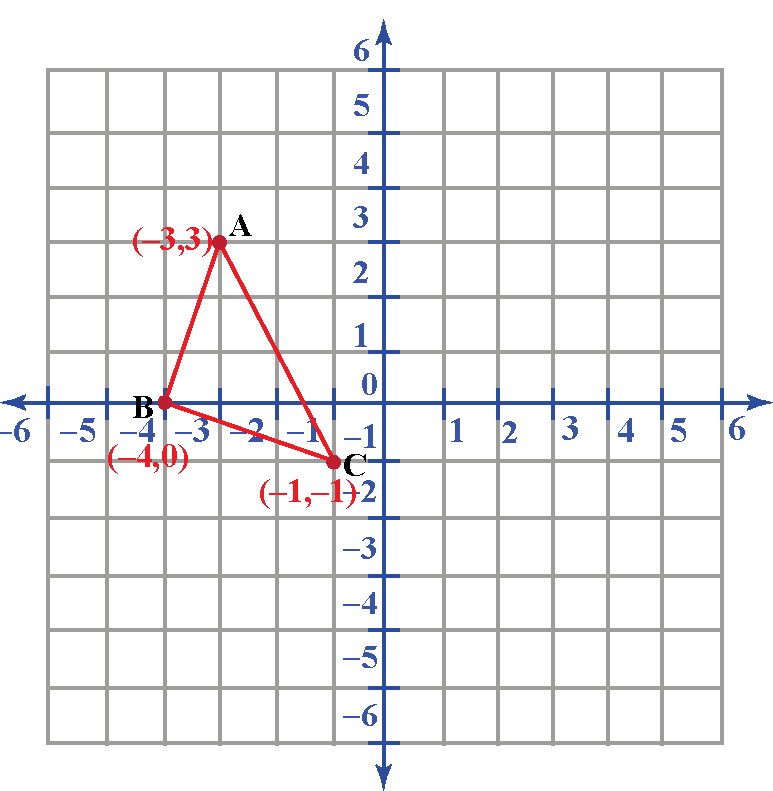The triangle is to be translated 2 units upward and 3 units toward the right.

The image after translation can be drawn as:Interactive Questions

Here are a few activities for you to practice.

Let's Summarize

The mini-lesson targeted the fascinating concept of translation math. The math journey around translation in math started with what a student already knows and goes on to creatively crafting a fresh concept in the young minds. Done in a way that not only it is relatable and easy to grasp, but also will stay with them forever.

At Cuemath, our team of math experts is dedicated to making learning fun for our favorite readers, the students!

Through an interactive and engaging learning-teaching-learning approach, the teachers explore all angles of a topic.

Be it worksheets, online classes, doubt sessions, or any other form of relation, it’s the logical thinking and smart learning approach that we, at Cuemath, believe in.

FAQs on Translation

1. What are rigid transformations?

A rigid transformation (also called an isometry) is a transformation of the plane that preserves length, which means that the object is transformed without getting its dimensions affected.

2. What are the 4 transformations?

There are four types of transformations possible for a graph of a function. They are:

• Rotations
• Translations
• Reflections
• Dilation

3. How do you solve translations?

Translation for any object can be solved in two sections, depending on whether the object is translated horizontally, vertically, or both.

A function, f(x), after translation can be represented in the general form as:

$$f(x) = f(x \pm k) \pm C$$

where,

• k is the number of units translated horizontally
• C is the number of units translated vertically

4. What do you mean by geometric transformation?

Geometric transformation of any plane means transformation that includes changes in its size, shape, and orientation.

More Important Topics
Numbers
Algebra
Geometry
Measurement
Money
Data
Trigonometry
Calculus
More Important Topics
Numbers
Algebra
Geometry
Measurement
Money
Data
Trigonometry
Calculus
Learn from the best math teachers and top your exams

• Live one on one classroom and doubt clearing
• Practice worksheets in and after class for conceptual clarity
• Personalized curriculum to keep up with school